# Novel Implementations of Clutter and Target Discrimination Using Threshold Skewness Method

Novel Implementations of Clutter and Target Discrimination Using Threshold Skewness Method
SENSE, VIT University, Vellore 632014, TamilNadu, India

Corresponding Author Email:
vijaykumar@vit.ac.in
Page:
1079-1085
|
DOI:
https://doi.org/10.18280/ts.380418
9 April 2021
|
Accepted:
25 July 2021
|
Published:
31 August 2021
| Citation

OPEN ACCESS

Abstract:

Now a day’s defence applications associated to novel, army and military war fields are required wall imaging discrimination. As of now many wall-imaging techniques are designed but cannot discriminate the target and clutter with accurate working. Therefore, a novel advance wall image tracking method is required for differentiate the clutter and human target. In this research work single value decomposition technique is used to estimate the range bin behind the wall target. In order to track the target and clutter single-value-decomposition (SVD) is not sufficient, so that along this SVD, threshold skewness (TS) method has been presented. Combination of SVD-TS giving the accurate long range-bin sensing and directed the human’s targets. SVD-TS method is a statistical scheme, which can realise the amplitude ranges through large number of range-bin scans. This technique improves the accuracy by 98.6%, skewness by 8%, and normalised power by 98.9%. These SVD-TS method is more efficient and compete with existed techniques.

Keywords:

SVD, TS, clutter, target

1. Introduction

The target and clutters are tracking through many algorithms, these are operated in uncertainty environment and origin of measurement. Many algorithms cannot accurately detect the targets, instead of them, they identify the wall clutters. This is happening due to unwanted scatters and measurement issues in the designed algorithms. The spatial and spectral image processing techniques are used for multidimensional estimating process. The dimensions of the converted square values are the parameters can be determined referring to in the field "singular value decomposition," and those values can tell you a number of things. If one of the singular values is 0, for illustration, this transformation will flatten the model. In this unbalanced and inverse Clutters has been identified over measurement optimisation technique. The multi target tracking technology implemented by adaptive spatial Cluttering estimation model. In this target are detected with riders or Sonars, these are detecting the human targets through the wall without Cluttering. Different algorithms are proposed for Cluttering and target measurements to achieve efficient and robust human target discrimination. The point of detection in special Cluttering computing identifies the exact human target at limited adaptive figuring.

In this research work singular value decomposition model is used to estimate the various range of human targets. The SVD technique is not a sufficient to approximate the target, it requires long range target identification with less power CLUTTER resulting. But, SVD consumes maximum powers results that CLUTTERs have been identified at less long range. Therefore, this method is not suitable for wall imaging human targets identification. The decision matrix is a different type of human target detection system it needs more information than available elements. To identify is the human targets attaining circumstances and long-range bin an advance decision system is compulsory. Therefore, threshold skewness decision matrix statistical model is implemented. It is a distributive process such as signal estimation, energy detection and received radar range CLUTTER estimation have been identified. This technique differentiates the target and CLUTTER with accurate mechanism. The combination of svd and threshold skewness model is a novel technique it can examine the target at long range without any disturbance.

The conventional models cannot provide the accurate solution for target identification at long range from wall. So statistical analysis is compulsory. To study the statistical and probabilistic long-range profiles can be providing the efficient and robust results at the time of target detecting. A, B and C are the three ranges, in this B is the wall, A is a target and C is sensing point. The target is easily identified when complete your profile applied on pre-processed data. Figure 1 explains about the range profile amplitudes due to breathing.

## 1.png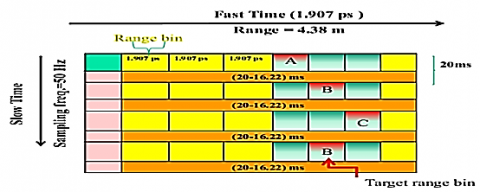Figure 1. An example of range profile amplitude changes due to breathing

The above method illustrated that double range profile amplitude system, in this target range bin has been detected with an accurate manner through in less time. The high signal amplitude targets are easily cross over and attenuated by band-pass filter. In this investigation 46 test samples are investigated and distributed for pre-processing in the various environmental conditions. All the attained results clearly shows that the distributions are squared after applying the proposed algorithm. These are identifies the results with long ranges than a target adjust. The CLUTTER and target distribution can be introduced by Weibull mechanism.

The correlation of probability distribution function has been estimated through P(x).

$P_{x}(x)=\frac{k}{\lambda}\left(\frac{x}{\lambda}\right)^{k-1} e^{-(x / \lambda)^{k}} x>0$                 (1)

The Eq. (1) clearly explains about probability estimation of SVD correlation. In this k>0 and lamda >0 represents that shape and scaling. The shape element is an important skew parameter for distributing the target in the sensing path. Coming to scaling parameter it is an inverse proportional function. The through wall imaging system is an emerging investigation for various civil and military applications.

2. Related Work

The civil warfare situations are extensively utilized for advanced developing countries. The wall imaging system mainly working on UWB radar . In this author can estimating radar based imaging technique thorough differentiated modelling.

Through the wall imaging technology is most helpful for defence applications as well as satellite applications. Many techniques for PWI are presented but they are giving less accurate results. For 100 factors eco signal system can only provide the 4dB peak to signal noise ratio .

The radar signaling for UWB implementation is performed for wall through imaging system, this can helpful for aerospace applications .

The goodness of fit approach is a linear regression model, in this wall images are easily targeted with random index element. The exact curve fitting and prediction components are providing the long-range bin target and CLUTTER detection. This method gives the 81.36% of throughput at 0.44mm bias in length .

The relay amplitude function and radar Cluttering models are advanced high resolution wall imaging techniques. In this normalized Weibull probability distributions have been provided for better square shape density detections. This statistical detection allowing the emerging efficient and accurate image tracking .

Table 1. Literature survey

The falconer utilizes the pulse Doppler based microwave radar, it is working UHF range of band. This study gives the investigation of Doppler discriminators among stationary, non-stationary and human targets . The chest of human and their motion has been detected behind the wall using statistical distribution method. In this experiment Gaussian distribution and essential PCA models are discussed . Rayleigh, K-, and Weibull distribution was planned for clutter  using X-band radar. The inactive Cluttering profile and its magnitude function as presented for normal log exponential model by Weibull, it is a non-stationary Cluttering process and identifies the moving targets at any normal situations. Weibull, Rayleigh distributions are presented in the studies [20, 23, 24]. To differentiate the stationary and non-stationary targets removal noise functionality mechanism has been proposed by kurposis . While more distributed noise and momentum of targets has easily shootout through pixels in the wall imaging function. By contrast adjustment in the selected image identifies the movable targets . The link among A in the k value and the correlation matrix's eigen vectors. The Principal Components of Data Matrices are the right singular verticals (V) of the provided matrix in the order of the Singular Values, because n is identical in both situations. Random selection sampling is a technique for selecting a smaller sample size from a larger group in order to do research and make generalisations about the bigger number. A selecting strategy in which each test has an equal chance of being selected is known as random selection. Each component of the set has an equal chance of being chosen as part of the sampling process in random selection. Random sampling in qualitative research is closer to what is known as "random assignment" in technical terms. Following a survey method that identifies a group of potentially useful data sources, the next step could be to employ random sample to determine which instances to investigate. Therefore, random sampling mechanism does not work for long range bin applications The organization of the paper is as follows section 1 introduces the paper section 2 gives information about fitting the clutter and target distributions in Weibull Distribution section 3 elaborates the Experimental evaluation along with results, section 4 briefly discusses about the paper and section 5 concludes the paper.

3. Weibull Clutter Distribution

The magnitude distribution of square of correlated in ultra-wideband detectors are investigated clearly. Richards  proposed that goodness of fit technique, it is an exact curve fitting model for imaging the wti chi squared test and kolmorgooy god test were implemented for identifies the clutter distribution and target. These two methods are more helpful for identifies the wall through image Weibull or not.

3.1 Chi squared test

Two perform the chi squared test there is random samples are required. Those are clearly shown $x_{1}, x_{2}, x_{3}, x_{4} \ldots \ldots x_{i}, \ldots x_{n}$ these are clearly explaining the distribution function and its certain approach. The many studies are performed random sample mechanism but these are not working for long range bin applications. So that singular value decomposition mechanism with threshold skewness method is proposed, where $i \in\{1: n\}$ to cells $A_{1}, A_{2}, \ldots . A_{j}, \ldots . A_{M} j \in\{1: M\}$ .

$p_{i}=p\left(x \in A_{j}\right)=\int_{A_{j}}^{n} d F(x)$        (2)

The Eq. (2) clearly explains about probability of loss samples and human target detection without any clutter. A loss that makes or enhances the risk of losing event. An insured's action that enhances the likelihood of a loss or the severity of a loss. Where p is the probability of x, this theory suggested that high dimensional tracking of human targets behind the wall through clutters. The statistical information gives the random variance and its specific distribution related to TWI.

$A^{2}=\sum_{i=1}^{M} \frac{\left(n_{i}-n_{p i}\right)^{2}}{n_{p i}}$         (3)

The Eq. (3) clearly explains about chi-squared statistical equation, in this A is the individual tracking function M is the number of available cells and N is the number of observations. Here X is the number of columns in the data sets and NP is the total number of observations.

3.2 KOLMORGOOV GOF test (KGOF):

The empirical function of distribution is a real time data set it continuously monitor the curve fitting in the wall through images. This study concentrates on specific distributions in the available real time data sets. The f(x) is the unknown distributor and it can measure the long-range bin distance through the radar. If this function cannot identify the distributor, then E.D.F. adjusted the level of distribution with two-dimensional nature .

$D=\max \left(D^{+}, D^{-}\right)$      (4)

The insights that measure the distinction among F(x) and F_n (x) are known as the EDF measurements . Two insights are D^+, which is equivalent to the biggest vertical distinction among F(x) and F_n (x) when F_n (x) is more prominent than F(x) , and D^-, which is equivalent to the biggest vertical contrast among F(x) and F_n (x) when F_n (x) is littler than F(x) . Kolmogorov presented the most notable measurement .

$F(x)=1-\exp \left\{-\left(\frac{x}{\lambda}\right)^{k}\right\} k \geq 0$              (5)

The KGOF test has been performed for Weibull distributions and these are converted to reliable data flows. It is an excellent and extreme valuable distribution function through this method can estimate the human targets with effective manner. Let $y=-\log (x)$. KGOF is an aggressive test which is implemented on MATLAB software. Here available continues data is converted into reliable discrete samples with streaming view.

$F(y)=\exp \left[-\exp \left\{-\frac{y-\varphi}{\theta}\right\}\right],-\infty<\infty$       (6)

where, $\theta=1 / k$ and $\varphi=-\log \lambda$ the new random distribution novel method implemented for target detection without any cluttering. In this research work 46 samples are taken as reference for measure the long-range target. The result of above test case gives the better accuracy and throughput. This KGOF method identifies shape and target easily. When we are using with this type of 2 test cases, we can measure the short- and long-range bin operations with effective manner. An empirical distribution (also known as an actual Continuous Distribution, eCDF) in statistical distribution is associated with a pattern's empirical measure. This sampling distribution is a smart system with each of the n pieces of data jumping up by 1/n.

4. Experimental Test Verification

In this SVD TS proposed method selecting the 46 samples with two persons. in this test case normal breathing operations standing operations can be performed at 3.5 metres away from UWB radar.

## 2.png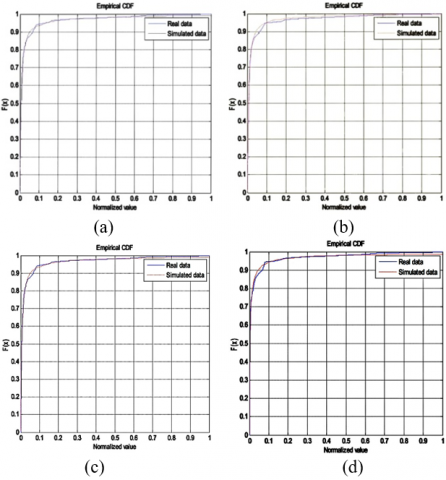Figure 2(a-d). CDF analysis

The thickness of the wall decided as 20cm made by gypsum material. In this one subject is common for all test cases that is the wall is selected as concrete. The check and square test cases was applied S magnitude output with correlator that was clearly shown in above mathematical competitions. the entire research work applied on column and row wise operations at clutters range bin and target range bin assumed 10 to 20 meters. The raw data clearly applied on proposed chi-squared test measurement as well as KSGOF test measurement whereas first method passed forty three samples and three are rejected. Coming to second method all 46 samples are detected with normal distribution. The fitted data can be distributed using shape factor via Weibull discriminator. The cumulative distribution function higher than the conventional method the normalisation easily applied on statistical data are getting the accurate output low complex correlation.

## 3.png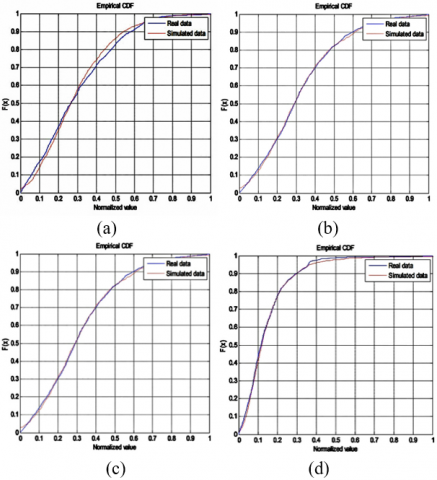Figure 3(a-d). CDF data

The Figure 2 explains about cumulative distribution function of normalised operation, it is clearly identified that different transmitted powers are estimated at -16 DB - 15 DB - 14 DB and -13 DB. These all output correlations gives the long-range bin fitness curves.

## 4.png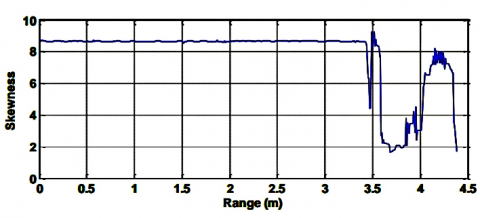Figure 4. Skewness analysis

Figure 3 clearly explains about cumulative distribution function of random square variable function. In this the four types of results are identified -16 DB -15 DB -14 DB and -13 DB results are shown clearly in the above explanation. Figure 4 explains about threshold skewness singular value decomposition technique this output is belonging to correlation at every range bin operation. The breathing operations are normalised at 3.5 metres from UWB radar. The measurements considered as 1.5 metres 20 metres behind the wall and gypsum concrete wall respectively. This curve decreases the skewness and gives the operation at less than 3. In the following above curve easily identified that cumulative distribution function of Weibull operations at KGOF method. In this 0.113 0.103 0.113 0.119 test cases were identified through threshold skew technique. At every case long range bin mechanisms are studied with values such as 0.044 0.032 0.020 and 0.038 respectively.

## 5.png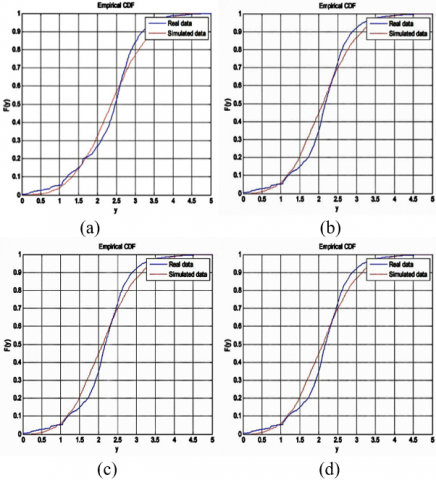Figure 5. CDF with SVD-TS

## 6.png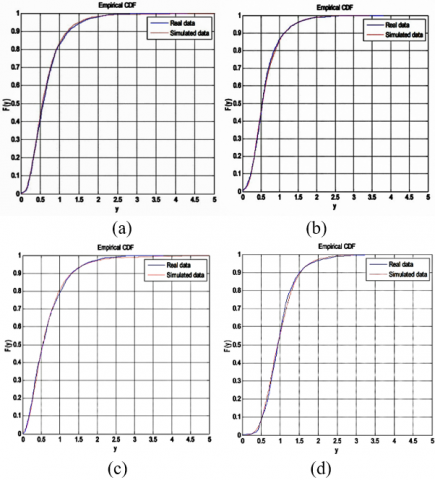Figure 6. CDF SVD-TS

Figures 5 & 6 clearly explain about magnitude squared functioning of proposed SVDTS mechanism. At all cases proposed method achieves good accuracy compared to existed methods.

Threshold $=\left[\operatorname{mean}\left(\left|\gamma_{i}\right|\right)-0.5 \sigma\right]$      (7)

The mean and variance values of Eq. (7) distributed that human targets are easily identified at various clustering places. Here y is the skew correlator, n is the normalised range bin Sigma is the skewness variance.

## 7a.png(a)

## 7b.png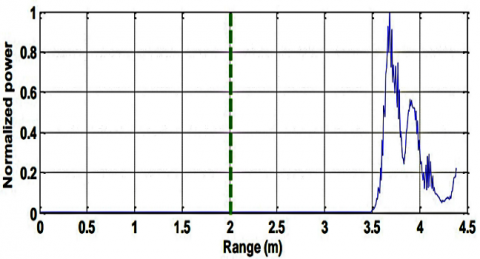(b)

## 7c.png(c)

## 7d.png(d)

Figure 7. CDF- after median filtering

$Skewness\begin{matrix} {{H}_{0}} \\ > \\ < \\ {{H}_{1}} \\ \end{matrix}Threshold$          (8)

Eq. (8) clearly explains about decision of target human body with skewness test. Here h0 is the hypothesis is null value clusters are existing. H1 is the hypothesis value when cluster and echoes continually applied on target. This case target is standing behind the 3.5 metres from the radar and 1.5 metres from the gypsum wall. The experiment is conducted on radar seeker laboratory of rci Hyderabad India. Figure 7 a b c shows that normalised operations of two thousand scams against the range bin operations. In this case without singular value decomposition results are getting low degradation.

## 8a.png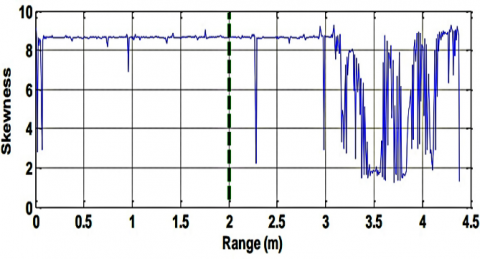(a)

## 8b.png(b)

## 8c.png(c)

## 8d.png(d)

Figure 8. CDF with normalized SVD-TS with median filtering

It is identified that the skewness values removing the echoes with median filter. In this case all 5 samples are neglected by SVD. Figure 7 demonstrated that output median filter with clear SCD values in this case target is clearly identified with exact location. If target standing behind the assumed concrete wall with proposed thickness the SVD and TS achieved better and accurate target identification.

The above Figure 8, a, b, c and d clearly explains about normalized scanned elements-2000 range of power. In this singular value decomposition and suppression techniques are critically scan the 2000 range of elements for normalized power. Here first singular value gives the skew-ness for continuous instances. The median filter gives the better observations without changing the scanned elements. In this investigation target bin range has been updated for every test element. The simulation results are independent on wall composition, this study also supporting the different types of mixture of wall such as gypsum and concrete. Some other experiments are performed on wood doors and brick wall. In this investigation proposed method explore the following limitations and detect the humans with various type of walls. To diminish the overlapping area between human targets and noise differentiate by distributive amplitude correlations. These are square type of correlation which can differentiate the target and disorders. This method increases the probability of detection and diminishes the false alarm rate. In this work increasing the signal to noise ratio on received signal, the signal to noise ratio improvement gives the target system detection possible with high accuracy. The amplitude squared distributed signal has been increases two curves apart from noise samples. This method decreases the overlapped area and improves the error rate, the alternative solution for following work is to reduce the variance and noise power . Coming to final solution for this problem the skewness method differentiates between curves. In this case adaptive thresholding method is not necessary. For thresholding value we are varying the skew-ness samples for sufficient range.

5. Discussion

In this research work SVD TS method is explained and investigated for estimating the human targets behind the wall. The principal component analysis gives the very slow time results with zero disturbance. But, two targets applied at a time this method is not qualifies the test cases. Therefore, SVDTS method qualifies all test conditions. The chi square test method and KGOF method achieves more accuracy and eye detection of targets at long range bins for discriminating of multiple targets.

6. Conclusion

In this research work a Rang bin values are disturbed via threshold skewness function. The squared function can easily identify the square of signal change with auto method. Existed method cannot be identified done long range bin distances. In order to track the target and clutter single-value-decomposition (SVD) is not sufficient, so that along this SVD, threshold skewness (TS) method has been presented. Combination of SVD-TS giving the accurate long range-bin sensing and directed the human’s targets. It was shown that fitting the probability distribution of square of the signal reflected from the clutter and human target to Weibull distribution passed the Chi-squared test and Kolmogorov-Smirnov test.

SVD-TS method is a statistical scheme, which can realise the amplitude ranges through large number of range-bin scans. This technique improves the accuracy by 98.6%, skewness by 8%, and normalised power by 98.9%. These SVD-TS method is more efficient and compete with existed techniques.

References

 Pardhu, T., Kumar, V. (2018). An investigation on human identification behind the wall. Journal of Advanced Research in Dynamical and Control Systems, 10(5): 122-129.

 Pardhu, T., Kumar, V. (2016). Reduction of clutter using TWI ultra wideband imaging. International Journal of Ultra-Wide Band Communications and Systems (IJUWBCS), 3(2): 101-106. https://doi.org/10.1504/IJUWBCS.2015.077142

 Pardhu, T., Kumar, V. (2016). Implementation of TWI using UWB radar signals. In the proceedings of International Conference on Recent Trends in Engineering, Science & Technology. https://doi.org/10.1049/cp.2016.1496

 D’agostino, R.B., Stephens, M.A. (1986). Goodness-of-Fit Techniques, Burnaby. Canada: Marcel Dekker, Inc.

 Shnidman, D.A. (1999). Generalized radar clutter model. IEEE Transactions on Aerospace and Electronic Systems, 35(3): 857-865. https://doi.org/10.1109/7.784056

 Richards, M.A. (2014). Fundamentals of Radar Signal Processing. McGraw-Hill Education.

 Yarovoy, A.G., Ligthart, L.P., Matuzas, J., Levitas, B. (2006). UWB radar for human being detection. IEEE Aerospace and Electronic Systems Magazine, 21(3): 10-14. https://doi.org/10.1109/MAES.2006.284354

 United States Geological Survey (USCG). (2013) Earthquakes with 50,000 or More Deaths. http://earthquake.usgs.gov/earthquakes/world/most_destructive.php.

 Gill, J., Moore, M.J. (2013). The State of Aging & Health in America. Centers for Disease Control and Prevention, Atlanta.

 Li, Z., Li, W., Lv, H., Zhang, Y., Jing, X., Wang, J. (2013). A novel method for respiration-like clutter cancellation in life detection by dual-frequency IR-UWB radar. IEEE Transactions on Microwave Theory and Techniques, 61(5): 2086-2092. https://doi.org/10.1109/TMTT.2013.2247054

 Zhang, Z., Zhang, X., Lv, H., Lu, G., Jing, X., Wang, J. (2012). Human-target detection and surrounding structure estimation under a simulated rubble via UWB radar. IEEE Geoscience and Remote Sensing Letters, 10(2): 328-331. https://doi.org/10.1109/LGRS.2012.2205555

 Mohamadi, F., Oijala, M., Zolghadri, M. (2013). U.S. Patent No. 8,358,234. Washington, DC: U.S. Patent and Trademark Office.

 Zhang, B., Wang, W. (2013). Through-wall detection of human being with compressed UWB radar data. EURASIP Journal on Wireless Communications and Networking, 2013(1): 1-7. https://doi.org/10.1186/1687-1499-2013-162

 Amin, M.G., Ahmad, F. (2013). Change detection analysis of humans moving behind walls. IEEE Transactions on Aerospace and Electronic Systems, 49(3): 1410-1425. https://doi.org/10.1109/TAES.2013.6557995

 Cuddihy, P.E., Ashe, J.M., Bufi, C.N., Genc, S. (2014). U.S. Patent No. 8,742,935. Washington, DC: U.S. Patent and Trademark Office.

 Lazaro, A., Girbau, D., Villarino, R. (2014). Techniques for clutter suppression in the presence of body movements during the detection of respiratory activity through UWB radars. Sensors, 14(2): 2595-2618. https://doi.org/10.3390/s140202595

 Lv, H., Li, W., Li, Z., Zhang, Y., Jiao, T., Xue, H. (2014). Characterization and identification of IR-UWB respiratory-motion response of trapped victims. IEEE Transactions on Geoscience and Remote Sensing, 52(11): 7195-7204. https://doi.org/10.1109/TGRS.2014.2309141

 Tivive, F.H.C., Bouzerdoum, A., Amin, M.G. (2011). An SVD-based approach for mitigating wall reflections in through-the-wall radar imaging. In 2011 IEEE RadarCon (RADAR), pp. 519-524. http://dx.doi.org/10.1109/RADAR.2011.5960591

 Amin, M.G. (2005). Radar, signal, and image processing techniques for through the wall imaging. In Digital Wireless Communications VII and Space Communication Technologies, 5819: 33-45. https://doi.org/10.1117/12.609921

 Haykin, S., Deng, C. (1991). Classification of radar clutter using neural networks. IEEE Transactions on Neural Networks, 2(6): 589-600. https://doi.org/10.1109/72.97936

 Falconer, D.G., Ficklin, R.W., Konolige, K.G. (2000). Robot-mounted through-wall radar for detecting, locating, and identifying building occupants. Proceedings 2000 ICRA. Millennium Conference. IEEE International Conference on Robotics and Automation. Symposia Proceedings (Cat. No.00CH37065), pp. 1868-1875. https://doi.org/10.1109/ROBOT.2000.844867

 Conte, E., De Maio, A., Galdi, C. (2004). Statistical analysis of real clutter at different range resolutions. IEEE Transactions on Aerospace and Electronic Systems, 40(3): 903-918. https://doi.org/10.1109/TAES.2004.1337463

 Shen, L., Kim, D.H., Lee, J.H., Kim, H.M., Park, P.J., Yu, H.K. (2011). Human detection based on the excess kurtosis in the non-stationary clutter enviornment using UWB impulse radar. In 2011 3rd International Asia-Pacific Conference on Synthetic Aperture Radar (APSAR), pp. 1-4. https://doi.org/10.1109/APMC.2013.6695005

 Lai, J.C.Y., Xu, Y., Gunawan, E., Chua, E.C.P., Maskooki, A., Guan, Y.L. (2010). Wireless sensing of human respiratory parameters by low-power ultrawideband impulse radio radar. IEEE Transactions on Instrumentation and Measurement, 60(3): 928-938. https://doi.org/10.1109/TIM.2010.2064370